This topic describes how to accelerate queries by sorting underlying data in AnalyticDB for PostgreSQL.

After you execute a `SORT <tablename>` statement, the system sorts the data of the specified table. Then, AnalyticDB for PostgreSQL pushes operators such as SORT down to the storage layer so that queries are accelerated based on the physical order of data. When your underlying data is ordered, your queries can be accelerated. This feature can accelerate queries that contain SORT, AGG, and JOIN operators based on sort keys.

Note
• The sorting acceleration feature requires all of your data to be ordered. After you write data, you must execute the `SORT <tablename>` statement again to order data.
• By default, the sorting acceleration feature is enabled.

## Example

In this example, a test table named far is used to compare the query time before and after sorting acceleration.

1. Execute the following statement to create a test table named far:
``````CREATE TABLE far(a int,  b int)
WITH (APPENDONLY=TRUE, COMPRESSTYPE=ZSTD, COMPRESSLEVEL=5)
DISTRIBUTED BY (a)  --Distribution key
ORDER BY (a);       --Sort key``````
2. Execute the following statement to write 1,000,000 rows of data to the far table:
``INSERT INTO far VALUES(generate_series(0, 1000000), 1);``
3. Execute the following statement to sort the data in the far table:
``SORT far;``

Query performance comparison:

Note The query time results in this example are for reference only. The query time varies based on various factors such as the data volume, computing resources, and network conditions.
• ORDER BY sorting acceleration
• Before sorting acceleration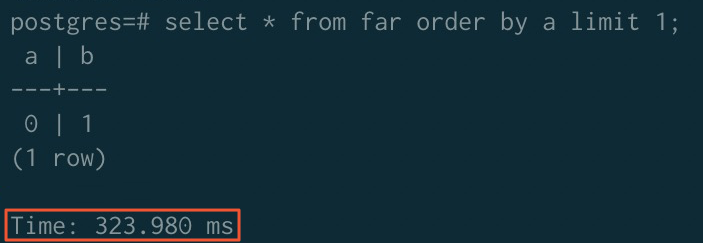• After sorting acceleration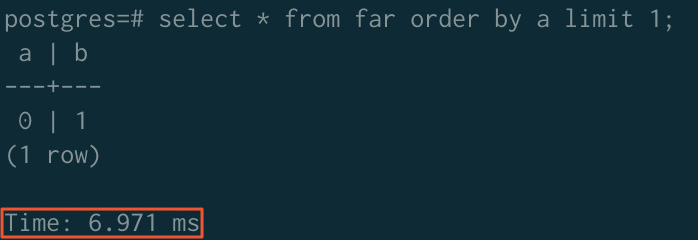• GROUP BY sorting acceleration
• Before sorting acceleration• After sorting acceleration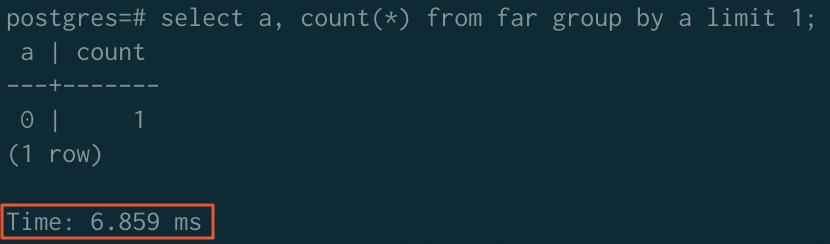• JOIN sorting acceleration
• Before sorting acceleration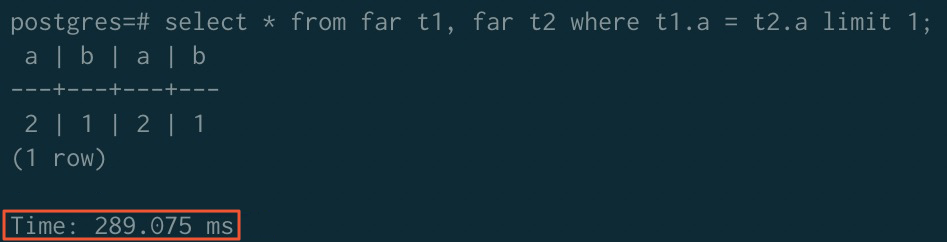• After sorting acceleration
Note If you want to use the sorting acceleration feature for JOIN operators, you must execute the following statements to disable the ORCA optimizer and enable the merge join algorithm:
``````SET enable_mergejoin TO on;
SET optimizer TO off;``````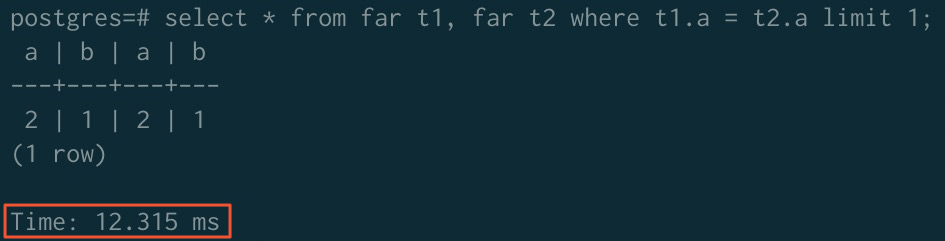- ORDER BY GROUP BY JOIN
Before acceleration 323.980 ms 779.368 ms 289.075 ms
After acceleration 6.971 ms 6.859 ms 12.315 ms